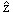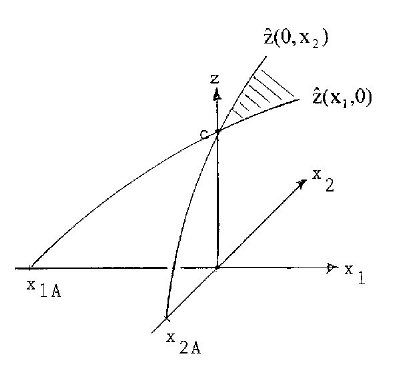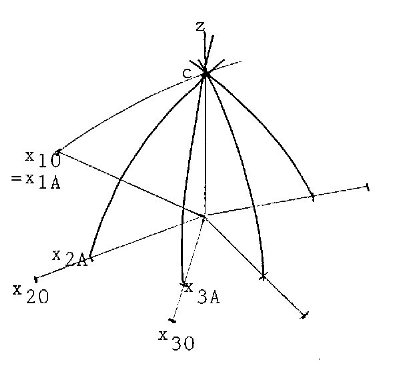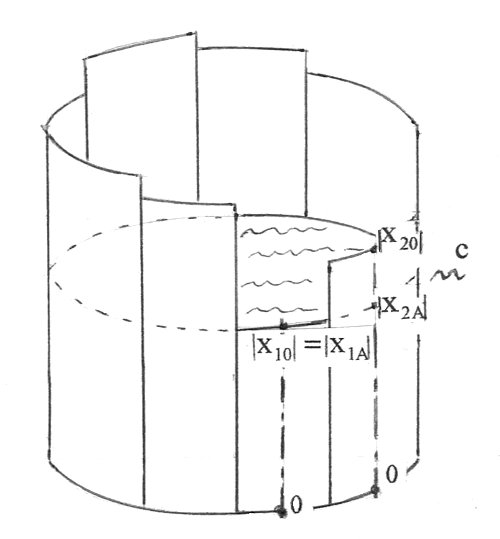# Liebig's Law: An Application of Generalized Mitscherlich's Law

## Abstract

Liebig's Law and his barrel-model are another presentation of the generalized Law of Mitscherlich. The staves of Liebig are not only of different length, but also with different scales.

## Introduction to Liebig's Barrel

The nowadays as Liebig's Law well-known fact originally was found by Carl Sprengel (1828); later popularized by Justus von Liebig (1803-1873). It says that only by increasing the amount of the limiting nutrient the crop-yield can be improved, independent of the other nutrients. The model of this is Liebig's barrel with the fertilizers as staves and the crop-yield as liquid - see the following figure 2.

It was shown in Mitscherlich's Generalized Law (Schneeberger, 2010b, paper 4), that the crop-yield(x1,.., xn) (for the hypothetical, z for the experimental crop-yield) as function of the n fertilizers x1,.., xn is the product of the one-dimensional functions(0,..xi,..0) multiplied by 1/cn-1, where c is the crop-yield for fertilizers xi=0. This was demonstrated with an example for n=2 in figures 3a and 3b and especially sketched in figure 2 of paper 4.

## Deduction of Liebig's Barrel

In figure 1a the essential parts of figures 2 and 3, paper 4, for n=2 fertilizers x1 and x2 are repeatedFigure 1a: Mitscherlich's curves(x1,0) and(0,x2); x1A=-0.376, x2A=-0.146Figure 1b: Generalization of figure 1

-xiA> 0 (i=1,2) are the quantities of fertilizer i, which yield crop=c, i.e. only with soil-immanent fertilizer. With n fertilizers we use the illustration in figure 1b. In general there will be -xi0 > -xiA >0, where -xi0 is the total quantity of soil-immanent fertilizer i, available in the soil for the experiment.

Figure 1b already suggests the idea of a cup, turned upside down, where the -xiA >0 are decisive for the contents of the cup. In Liebig's model the xi-axes are broadened to stoves of a barrel of length -xi0>0, the liquid (=crop-yield) c comes up to -xiA ≤ -xi0 of stave i. This means, that the scale is different for different staves. In example of figure 1a the liquid c reaches -xiA=0.376 at stave 1, but -x2A=0.146 at stave 2.

We now assume in figure 1b: x1A=x10, i.e. the whole available fertilizer 1 is spent for the crop. Then the limit of the liquid c is given by the limit of the stave 1 - see figure 2.Figure 2: Liebig's barrel

## References

Schneeberger, H. (2010b). Mitscherlich's Law: Generalization with several Fertilizers. Internet: http://www.soil-statistic.de, paper 4